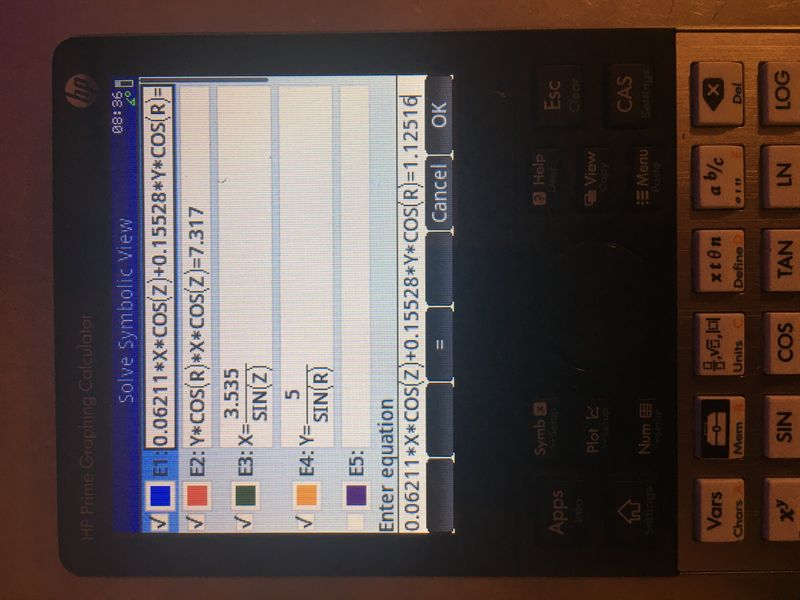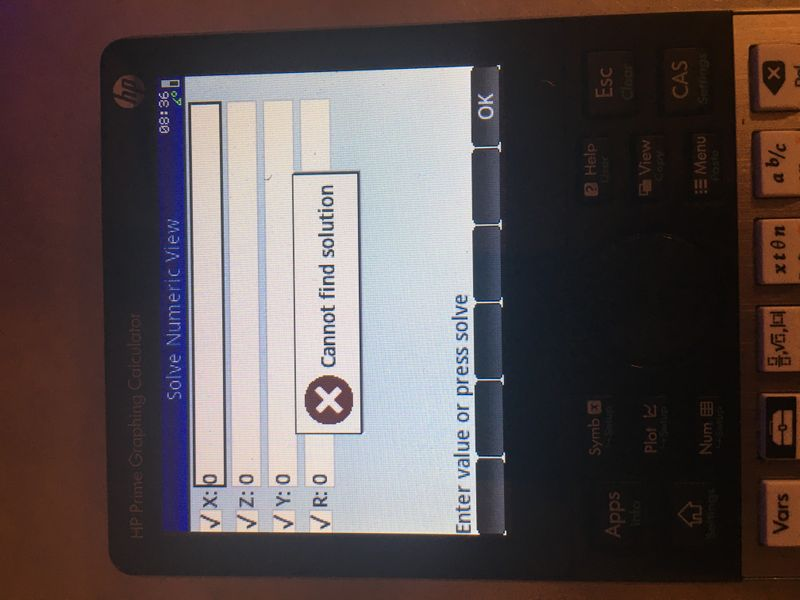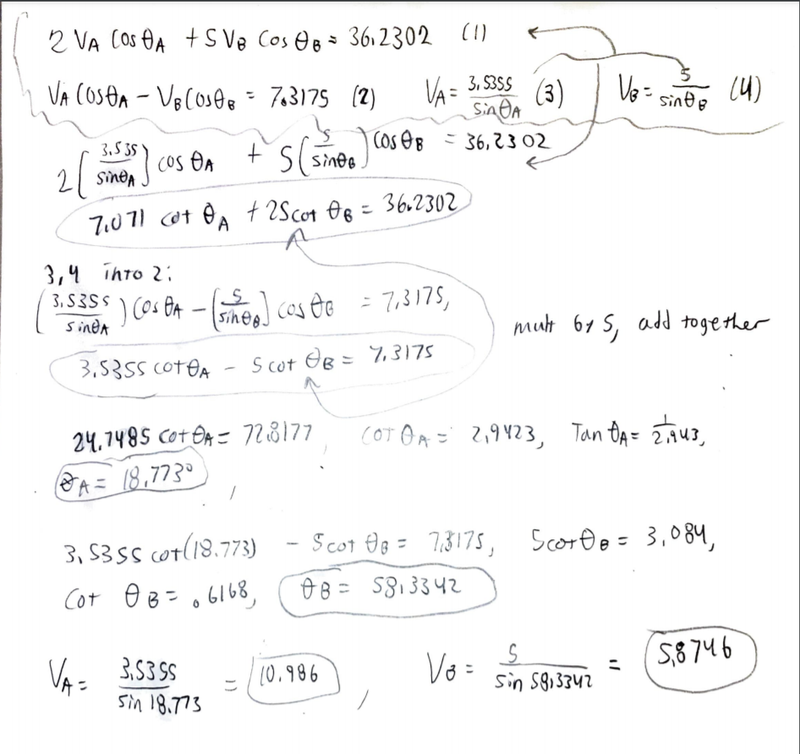cancel
Showing results for
Search instead for
Did you mean:New member
3 2 0 0
Message 1 of 5
299
Flag Post

# HP Prime - Solving 4 equations with trig functionsHP Recommended

Hello,

I have encountered some engineering problems that require solving for four variables (two velocities and two angles), and being an engineering course and not a math course, we are permitted a CAS to speed up the process. Attached is the equations as entered to the HP Prime G2, the error, and the work as done by hand. The scan has at the top, circled in the squiggly line, the equations I plugged in, then below the work.

I was wondering if there is a way to get these equations to work with the calculator.Work done by hand, squiggly line up top denotes equations to be inserted to CAS

4 REPLIES 4New member
3 2 0 0
Message 2 of 5
Flag PostHP Recommended

Are the pictures not showing up for others? If so I can probably toss em on IMGURLevel 8
622 609 117 207
Message 3 of 5
Flag PostHP Recommended

Prime's CAS is more powerful than the Solve app.  Try typing the following into CAS:

fsolve([(0.06211*x*cos(z)+0.15528*y*cos(r)) = 1.12516,(y*cos(r)*x*cos(z)) = 7.317,x = 3.535/sin(z),y = 5/sin(r)],[x,y,z,r])

This yields the following solution in degree mode:

x = −3.69437562108

y  = 8.45380808204

z = 253.109137022

r = 36.2599319908

That doesn't seem to be the solution that you reached, so I may have introduced a typo somewhere.  But the bottom line is that I suggest trying some of the more powerful solvers offered by Prime's CAS.

Disclaimer: I don't work for HP. I'm just another happy HP calculator user.

-Joe-New member
3 2 0 0
Message 4 of 5
Flag PostHP Recommended

Thanks, I was wondering how you typed the [x,y,z,r] bit. Is it shift, 5, put letters in? I tried in regular solve and it had a context error so I'm unsure if that's cause I wasn't using numerical solve or because of something else.Level 8
622 609 117 207
Message 5 of 5
Flag PostHP Recommended

Yes, [x,y,z,r] is typed into CAS by pressing Shift 5, then the letters with commas between them.  Be sure you're in CAS view, not in Home view or in an app, because lowercase variables which don't already exist will cause errors in Home and in the Solve app.

-Joe-
† The opinions expressed above are the personal opinions of the authors, not of HP. By using this site, you accept the Terms of Use and Rules of Participation